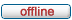# forum.alglib.net

ALGLIB forum
 It is currently Wed Oct 04, 2023 7:54 am

 All times are UTC

### Forum rules

1. This forum can be used for discussion of both ALGLIB-related and general numerical analysis questions
2. This forum is English-only - postings in other languages will be removed.

 Print view Previous topic | Next topic
Author Message
 Post subject: unexpected values in covariance matrix. lsfitlinearwPosted: Mon Nov 16, 2020 3:30 pmJoined: Tue May 08, 2018 3:15 pm
Posts: 5
Good day all!

I am using alglib-3.16.0.csharp.

There are relatively simple equations to solve unweighted linearly
least square problem Y = X * b:
b = (XT * X)-1 * XT * Y
covariance matrix for parameters b is M = RSS / ( n - 2) * (XT * X)-1. RSS - Residual Sum of Squares. The numerator, n−p, is the statistical degrees of freedom. In case for line n - 2.

and for the weighted linearly least square
b = (XT * W * X)-1 * XT * W * Y, where W - diagonal matrix
covariance matrix for parameters b is M = (XT * W * X)-1

In both cases, the variance of the parameter bi is given by Sqrt(Mii).

https://en.wikipedia.org/wiki/Weighted_least_squares

For unweighted case Alglib's results are equal whith results from equations before: parameters b and covariance matrix M. Сomplete match.
But for weighted case only results for parameters b are equal whith results from equations before. The covariance matrix, in turn, differs from the equation above.

Can someone tell me what's the matter?

//------------------------------------------------------------------------------------------------------------------------------------------
// data points Y
double[] y = new double[] { 62, 55, 59, 70, 67, 68, 65, 56, 83, 49, 60, 59, 74, 61, 57, 67, 55, 62, 67, 63, 65, 64, 66, 50, 66, 55, 62, 74, 63, 62, 63, 53, 70, 60, 55, 65, 69, 58, 61, 59, 67, 58, 52, 66, 46, 55, 62, 60, 56, 51 };

// data points X from 0 to 49.

double[,] x = new double[y.Length, 1];

for (int i = 0; i < y.Length; i++)
{
x[i, 0] = (double)(i);
}

// data points will be fitted by line function: Y = c + c * X

// Fmatrix forming

double[,] fmatrix = new double[y.Length, 2];

// all fmatrix[i, 0] = 1. This is for c

for (int i = 0; i < y.Length; i++)
{
fmatrix[i, 0] = 1;
}

// fmatrix[i, 1] = X[i]. This is for c

for (int i = 0; i < y.Length; i++)
{
fmatrix[i, 1] = x[i, 0];
}

int info;
double[] c;
alglib.lsfitreport rep;

//
// Linear fitting without weights
//
alglib.lsfitlinear(y, fmatrix, out info, out c, out rep);
System.Console.WriteLine("{0}", info); // EXPECTED: 1
System.Console.WriteLine("{0}", alglib.ap.format(c, 4));

//
// Linear fitting with individual weights.
//
//

// in my case variance(Y[i]) = Y[i], so I combine vector w[i] as
double[] w = new double[y.Length];
for (int i = 0; i < y.Length; i++)
{
w[i] = Math.Sqrt(1 / y[i]);
}

alglib.lsfitlinearw(y, w, fmatrix, out info, out c, out rep);
System.Console.WriteLine("{0}", info); // EXPECTED: 1
System.Console.WriteLine("{0}", alglib.ap.format(c, 4));

//-----------------------------------------------------------------------------------------------------------

Best regards

 Attachments:Alglib, MathCad, Me 2.png [ 422.09 KiB | Viewed 1574 times ]
TopPost subject: Re: unexpected values in covariance matrix. lsfitlinearwPosted: Fri Nov 20, 2020 1:43 pmJoined: Tue May 08, 2018 3:15 pm
Posts: 5
I have done a little research and can answer my own question.
Y = X * b
parameters with minimal RSS: b = (XT * X)-1 * XT * Y
covariance matrix for parameters b: exact form M = (XT * X)-1 * X * K * X * (XT * X)-1, where K is covariance matrix for Y.
But if you do not know K and suppose that Kii = sigma^2 (homoscedastic case, K = sigma^2 * I, M = (XT * X)-1 * XT * K * X * (XT * X)-1 = sigma^2 * (XT * X)-1 * XT * I * X * (XT * X)-1 = sigma^2 * (XT * X)-1) than you could calculate a tally for sigma^2 from RSS: sigma*^2 = RSS / ( n - 2).
So a tally M = RSS / ( n - 2) * (XT * X)-1. RSS - Residual Sum of Squares. The numerator, n−p, is the statistical degrees of freedom. In case for line n - 2.

For weighted least square the same is true.
You know about heteroscedasticity in your data. You choose W matrix, which in ideal case is equal to K-1.
b = (XT * W * X)-1 * XT * W * Y and covariance matrix for parameters b is M = (XT * W * X)-1.
If you do no know the ecxact form of K, but have some good tally for K (or you know relation between errors for Yi) you can transform the original equation (Y = X * b) into a new homoscedastic one by multiplying by the W matrix.
And the new equation Y_ = X_ * b will have the same solution (b) and like before (for OLS) you can tally M matrix: M = RSS / ( n - 2) * (X_T * X_)-1.

Thus alglib evaluates the covariance matrix from the data itself. This is the typical way for a computing software.

p.s. alglib do the same for none-linear least square problem (evaluates the covariance matrix from the data itself), I cheked it.
If you want tot use your tally for K-matrix not only to solve equation but rather for covariance matrix of parameters evaluation you need calculate M by yourself.

TopDisplay posts from previous: All posts1 day7 days2 weeks1 month3 months6 months1 year Sort by AuthorPost timeSubject AscendingDescending

 All times are UTC

#### Who is online

Users browsing this forum: No registered users and 1 guest

 You cannot post new topics in this forumYou cannot reply to topics in this forumYou cannot edit your posts in this forumYou cannot delete your posts in this forumYou cannot post attachments in this forum

Search for: